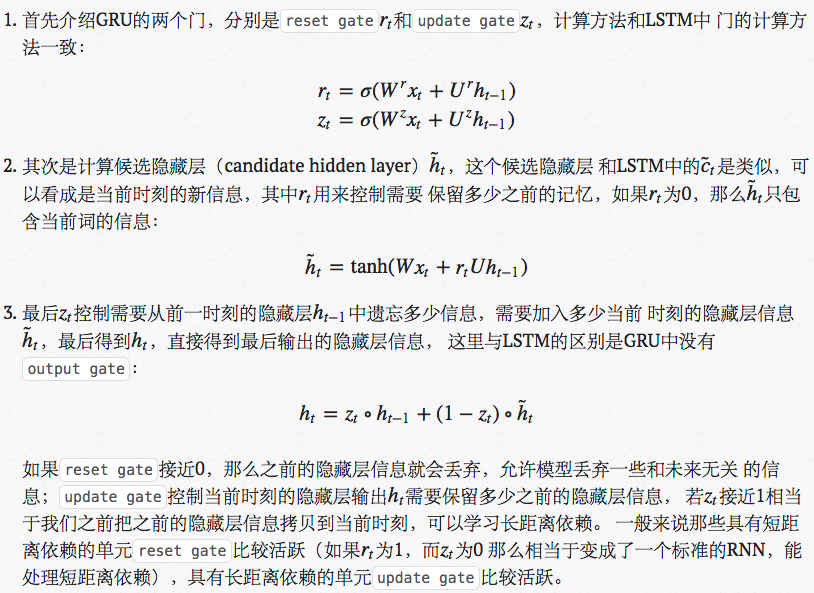# 递归神经网络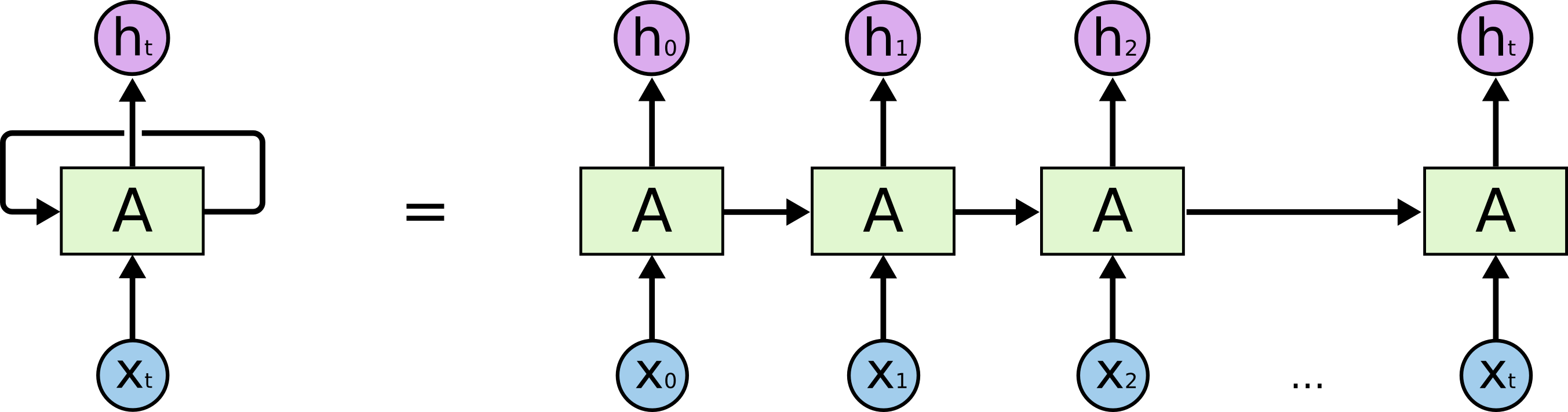## Series序列数据

RNN主要解决序列数据的处理，比如文本、语音、视频等等。这类数据的样本间存在顺序关系，每个样本和它之前的样本存在关联。比如说，在文本中，一个词和它前面的词是有关联的；在气象数据中，一天的气温和前几天的气温是有关联的。一组观察数据定义为一个序列，从分布中可以观察出多个序列。

$$P(X)=\prod_{i=1}^N{P(x_i|x_1,\ldots,x_{i-1})}$$

$$P(X)=\prod_{i=1}^N{P(x_i|x_{i-1},\ldots,x_{i-(k-1)})}$$

$$P(X)=\prod_{i=1}^N{P(x_i|x_{i-1})}$$

$$P(x_1, \ldots, x_T, h_1, \ldots, h_T, \theta) = P(h_1) \prod^T_{t=2}{P(h_t|h_{t-1})} \prod^T_{t=1}{(x_t|h_t)}$$

## RNN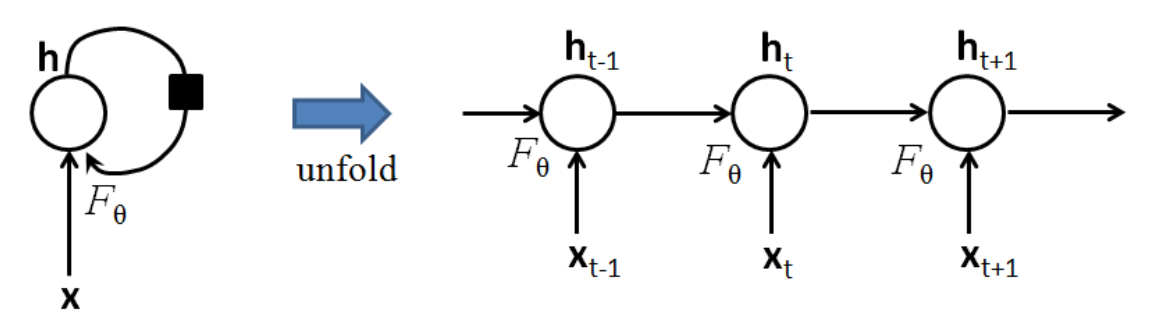$$h_t = F_{\theta}(h_{t-1}, x_t)$$

## RNN变种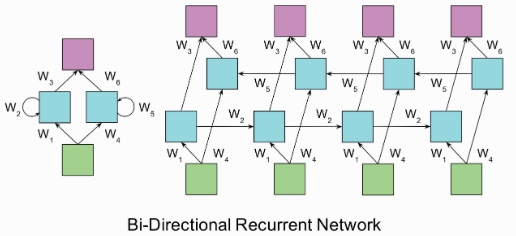Deep RNN

Deep(Bidirectional)RNNs与Bidirectional RNNs相似，只是对于每一步的输入有多层网络。这样，该网络便有更强大的表达与学习能力，但是复杂性也提高了，同时需要更多的训练数据。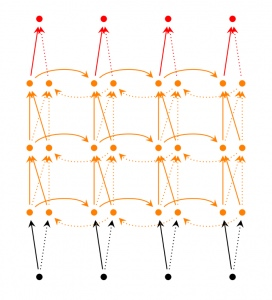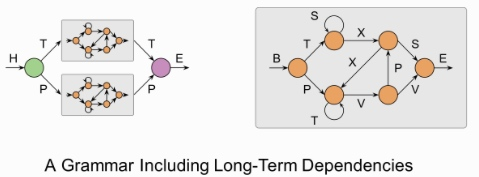RNN训练困难的主要原因在于隐藏层参数$$w$$的传播：由于误差传播在展开后的RNN上，无论在前向传播过程还是在反向传播过程中$$w$$都会乘上多次，这就导致：

• 梯度消失：如果梯度很小的话（<1），乘上多次指数级下降，对输出几乎就没有影响了
• 梯度爆炸：反过来，如果梯度很大的话，乘上多次指数级增加，又导致了梯度爆炸1. 有效初始化+ReLU激活函数能够得到较好效果
2. 算法上的优化，例如截断的BPTT算法。
3. 模型上的改进，例如LSTM、GRU单元都可以有效解决长期依赖问题。
4. 在BPTT算法中加入skip connection，此时误差可以间歇的向前传播。
5. 加入一些Leaky Units，思路类似于skip connection

## LSTM

LSTM 全称叫 Long Short-Term Memory networks，它和传统 RNN 唯一的不同就在与其中的神经元（感知机）的构造不同。传统的 RNN 每个神经元和一般神经网络的感知机没啥区别，但在 LSTM 中，每个神经元是一个“记忆细胞”（元胞状态，Cell State），将以前的信息连接到当前的任务中来。每个LSTM细胞里面都包含

• 输入门（input gate）: 一个Sigmoid层，观察$$h_{t-1}$$和$$x_t$$，对于元胞状态$$c_{t-1}$$中的每一个元素，输出一个0~1之间的数。1表示“完全保留该信息”，0表示“完全丢弃该信息”：

$$f_t=\sigma(W^fx_t+U^fh_{t-1})$$

• 遗忘门（forget gate): 一个Sigmoid层决定我们要更新哪些信息，并由一个tanh层创造了一个新的候选值（结果在$$(-1, 1)$$范围）

$$i_t=\sigma(W^ix_t+U^ih_{t-1})$$

$$\tilde{c}t=\tanh(W^cx_t+U^ch{t-1})$$

$$c_t=f_t\circ c_{t-1} + i_t\circ\tilde{c}_t$$

• 输出门（output gate）：控制哪些信息需要输出

$$o_t=\sigma(W^ox_t+U^oh_{t-1})$$

$$h_t=o_t\circ\tanh(c_t)$$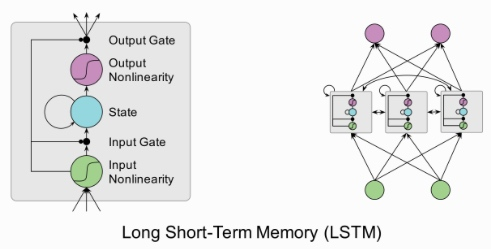• 模仿保罗·格雷厄姆进行写作
• 生成维基百科的 markdown 页面
• 手写识别
• 代码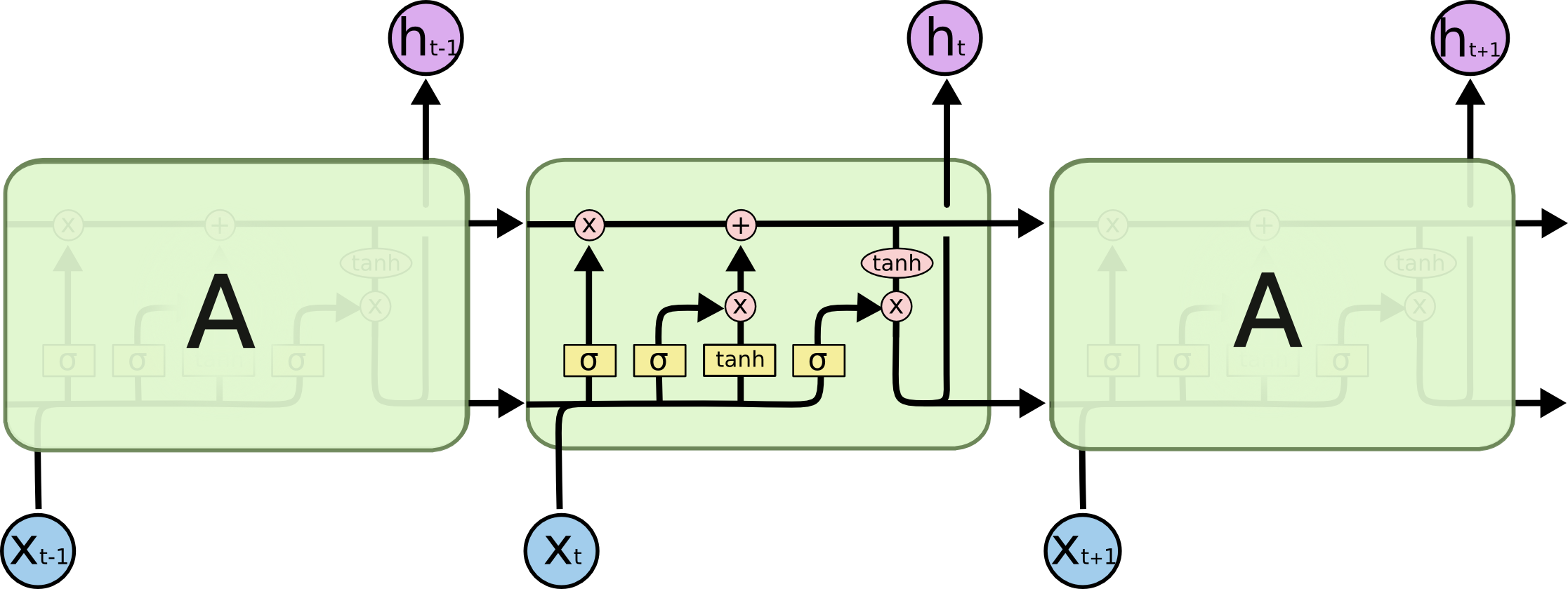### GRU

GRU (Gated Recurrent Unit) 是LSTM的变种，把LSTM中的遗忘门和输入门合并成为单一的“更新门(Update Gate)”，同时也将元胞状态(Cell State)和隐状态(Hidden State)合并，在计算当前时刻新信息的方法和LSTM有所不同。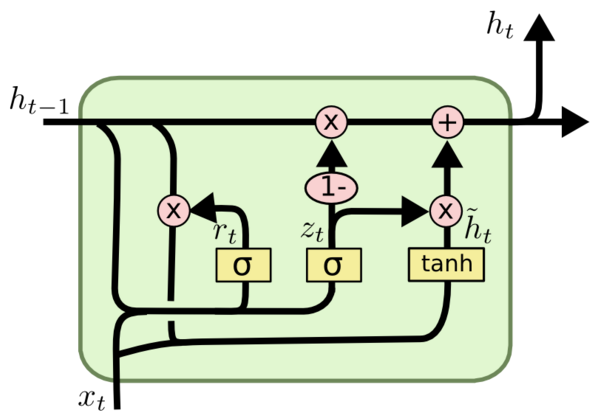#### GRU与RNN对比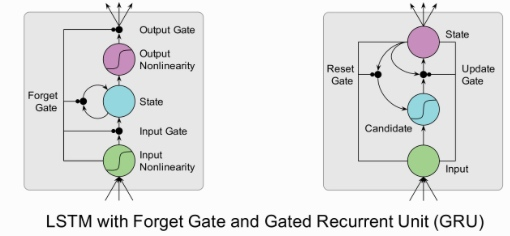#### GRU更新过程